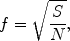Quantum dots (QDs) are potentially used for high-efficiency laser devices . It is crucial to control QD formation to arrange QDs with high uniformity and high density. Little is known, however, of the growth mechanism of QDs, in particular the surface reconstruction of a wetting layer (WL) and QD nucleation sites in Stranski-Krastanow (S-K) mode. Because the surface reconstruction changes microscopically and dynamically in the course of WL growth, an in situ scanning tunneling microscopy (STM) technique such as STMBE  is essential. Atomic-scale in situ observation of an InAs WL on a GaAs(001) substrate has revealed that the surface reconstruction of the InAs WL changes from c(4 × 4) to the mixture of (1 × 3)/(2 × 3) and (2 × 4) prior to QD formation . It is considered that such surface reconstructions form domains on InAs WL, and investigating their distribution will give a clue to understand a QD nucleation mechanism.

The distribution of reconstruction domains is characterized by spatial point patterns: a regular (ordered) pattern, a Poisson (random) pattern, and a clustered (aggregated) pattern . In a regular pattern, points are distributed uniformly. Voronoi tessellation, that is a polygonal decomposition of a space by perpendicular bisector lines among neighboring points, is often used in spatial point analysis. The standard deviation of Voronoi cell areas represents well the point patterns. For more precise analysis, the distance to the nearest neighbor point from an arbitrary position, r 1, is helpful . Let p(t) denote the probability that r 1 occurs less than t. The nearest neighbor distance function p(t) is identical to the probability of plotting a random point within the union area of circles whose radii are t and centers are the points. Trend of p(t) represents the characteristics of spatial point patterns.

In this paper, we investigate the surface reconstruction domains on InAs WL preceding QD formation by using in situ STM observation and discuss their distribution using spatial point analysis.

A piece (11 × 13 × 0.6 mm3) of GaAs(001) crystal was used as a substrate. First, the surface was thermally cleaned to remove the oxide layer under 1 × 10−4 Pa of an arsenic atmosphere in an MBE growth chamber. Next, a GaAs buffer layer was grown on the surface by using MBE until atomically smooth surface was obtained. The substrate was annealed at 430°C for 0.5 h to confirm the formation of c(4 × 4) reconstruction with reflection high-energy electron diffraction (RHEED). An STM unit was transferred to the sample holder in the growth chamber. A flux of In was irradiated to the sample during STM observation. After 1.5 monolayer (ML) of InAs WL growth, the substrate temperature was decreased to 300°C, and the As4 flux was shut off.

Figure 1 shows the STM image of 1.5 ML of InAs WL just prior to QD formation at 300°C. Stripes due to As dimers were clearly observed. The image was divided by a 25 × 25 mesh. The pitch of the As stripes, corresponding to the unit cell length along  azimuth of InAs surface reconstructions, was measured from the STM line profile for each cell. The data are plotted in the color diagram of Fig. 2. The pitch was classified into three ranges, namely the range from 0.6 to 1.0 nm, the range from 1.0 to 1.4 nm assuming (1 × 3)/(2 × 3), and the range from 1.4 to 2.0 nm assuming (2 × 4). Most of the cells had (1 × 3)/(2 × 3) or (2 × 4) surface reconstruction. Four neighboring cells having the same surface reconstruction were located in the diagram as indicated by oval markers in Fig. 3. A set of these cells correspond to a surface reconstruction domain extending for 16 nm2. For each of (1 × 3)/(2 × 3) and (2 × 4) surface reconstructions, the center points of the domains were marked, and their coordinates were measured by using ImageJ software [8, 9]. The center coordinates were used for the Voronoi tessellations of the STM view field (Fig. 4) and the computation of the nearest neighbor distance function p(t) . The cells touching the frame of the STM image was not used for the computation since they are not true Voronoi cells. For the calculation of p(t), t was normalized by the factor f as follows:where S is the total area of Voronoi cells, which are not touching the frame, and N is the number of valid reconstruction domains.